# Partial derivative

Jump to: navigation, search

## Definition at a point

### Generic definition

Suppose$f$ is a function of more than one variable, where$x$ is one of the input variables to$f$. Fix a choice$x = x_0$ and fix the values of all the other variables. The partial derivative of$f$ with respect to$x$, denoted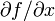$\partial f/\partial x$, or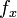$f_x$, is defined as the derivative at$x_0$ of the function that sends$x$ to$f$ at$x$ for the same fixed choice of the other input variables.

### For a function of two variables

Suppose$f$ is a real-valued function of two variables$x,y$, i.e., the domain of$f$ is a subset of$\R^2$. We define the partial derivatives as follows:

• Partial derivative with respect to$x$: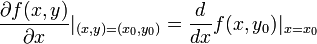$\frac{\partial f(x,y)}{\partial x}|_{(x,y) = (x_0,y_0)} = \frac{d}{dx}f(x,y_0)|_{x = x_0}$

In words, it is the derivative at$x = x_0$ of the function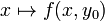$x \mapsto f(x,y_0)$.

This partial derivative is also denoted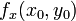$f_x(x_0,y_0)$ or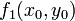$f_1(x_0,y_0)$.

• Partial derivative with respect to$y$: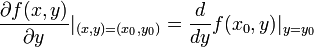$\frac{\partial f(x,y)}{\partial y}|_{(x,y) = (x_0,y_0)} = \frac{d}{dy}f(x_0,y)|_{y = y_0}$

In words, it is the derivative at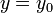$y = y_0$ of the function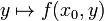$y \mapsto f(x_0,y)$.

This partial derivative is also denoted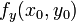$f_y(x_0,y_0)$ or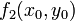$f_2(x_0,y_0)$.

### For a function of multiple variables

The notation here gets a little messy, so read it carefully. We consider a function$f$ of$n$ variables, which we generically denote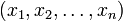$(x_1,x_2,\dots,x_n)$ respectively. Consider a point Failed to parse (PNG conversion failed; check for correct installation of latex and dvipng (or dvips + gs + convert)): (a_1_a,2,\dots,a_n) in the domain of the function. In other words, this is a point where$x_1 = a_1,x_2 =a_2, \dots, x_n = a_n$.

Suppose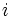$i$ is a natural number in the set$\{ 1,2,3,\dots,n \}$.

The partial derivative at this point$(a_1,a_2,\dots,a_n)$ with respect to the variable$x_i$ is defined as the derivative: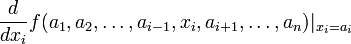$\frac{d}{dx_i}f(a_1,a_2,\dots,a_{i-1},x_i,a_{i+1}, \dots,a_n)|_{x_i = a_i}$

In other words, it is the derivative (evaluated at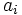$a_i$) of the function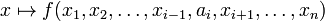$x \mapsto f(x_1,x_2,\dots,x_{i-1},a_i,x_{i+1},\dots,x_n)$ with respect to$x_i$, evaluated at the point$x_i = a_i$.